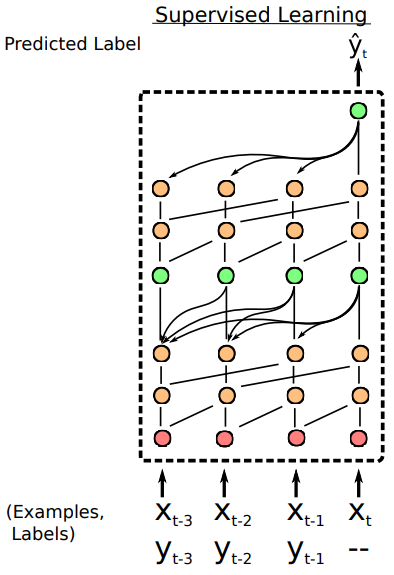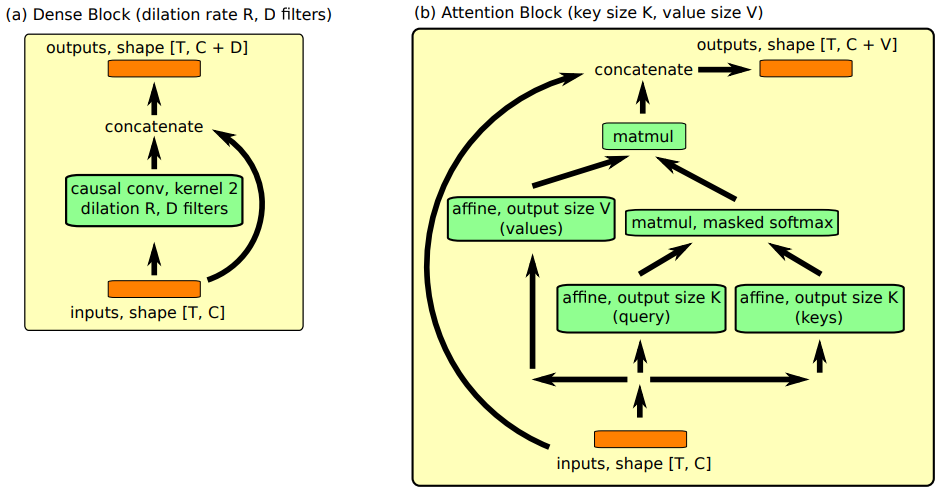# Simple Neural Attentive Learner¶

Simple Neural Attentive Learner (SNAIL) 组合时序卷积和 soft-attention， 前者从过去的经验整合信息，后者精确查找到某些特殊的信息。

## 1 Preliminaries¶

### 1.1 时序卷积和 soft-attention¶

soft-attention 可以实现从超长的序列内容中获取准确的特殊信息。 它将上下文作为一种无序的关键值存储，这样就可以基于每个元素的内容进行查询。 但是，位置依赖的缺乏（因为是无序的）也是一个缺点。

TCN 和 soft-attention 可以实现功能互补: 前者提供高带宽的方法，代价是受限于上下文的大小，后者可以基于不确定的可能无限大的上下文提供精准的提取。 因此，SNAIL 的构建使用二者的组合：使用时序卷积去处理用注意力机制提取过的内容。 通过整合 TCN 和 attention，SNAIL 可以基于它过去的经验产出高带宽的处理方法且不再有经验数量的限制。 通过在多个阶段使用注意力机制，端到端训练的 SNAIL 可以学习从收集到的信息中如何摘取自己需要的信息并学习一个恰当的表示。

### 1.2 Meta-Learning¶

$\pi\left(a_{t} \mid x_{1}, \ldots, x_{t} ; \theta\right)$

\begin{split} \begin{aligned} &\min _{\theta} \mathbb{E}_{\mathcal{T}_{i} \sim \mathcal{T}}\left[\sum_{t=0}^{H_{i}} \mathcal{L}_{i}\left(x_{t}, a_{t}\right)\right] \\ &\text { where } x_{t} \sim P_{i}\left(x_{t} \mid x_{t-1}, a_{t-1}\right), a_{t} \sim \pi\left(a_{t} \mid x_{1}, \ldots, x_{t} ; \theta\right) \end{aligned} \end{split}

## 2 SNAIL¶

### 2.1 SNAIL 基础结构¶### 2.2 Modular Building Blocks¶Densen Block 用了一个简单的因果一维卷积（空洞卷积）， 其中膨胀率 (dilation)为 $$R$$ 和卷积核数量 $$D$$ （ 对于所有的实验中设置卷积核的大小为2)， 最后合并结果和输入。 在计算结果的时候使用了一个门激活函数。 具体算法如下:

1. function DENSENBLOCK (inuts, dilation rate $$R$$, number of filers $$D$$):

1. xf, xg = CausalConv (inputs, $$R$$, $$D$$), CausalConv (inputs, $$R$$, $$D$$)

2. activations = tanh (xf) * sigmoid (xg)

3. return concat (inputs, activations)

TC Block 由一系列 dense block 组成，这些 dense block 的膨胀率$$R$$ 呈指数级增长，直到它们的接受域超过所需的序列长度。具体代码实现时，对序列是需要填充的为了保持序列长度不变。具体算法如下：

1. function TCBLOCK (inuts, sequence length $$T$$, number of filers $$D$$):

1. for i in $$1, \ldots, \left[log_2T\right]$$ do 1. inputs = DenseBlock (inputs, $$2^i$$, $$D$$)

2. return inputs

Attention Block  中设计成 soft-attention 机制， 公式为：

$\mathrm{ Attention }(Q, K, V)=\mathrm{softmax}\left(\frac{Q K^{T}}{\sqrt{d_{k}}}\right) V$
1. function ATTENTIONBLOCK (inuts, key size $$K$$, value size $$V$$):

1. keys, query = affine (inputs, $$K$$), affine (inputs, $$K$$)

2. logits = matmul (query, transpose (keys))

3. probs = CausallyMaskedSoftmax ($$\mathrm{logits} / \sqrt{K}$$)

4. values = affine (inputs, $$V$$)

5. read = matmul (probs, values)

## 3 SNAIL 分类结果¶

Method

5-way 1-shot

5-way 5-shot

20-way 1-shot

20-way 5-shot

Santoro et al. (2016)

82.8 $$\%$$

94.9 $$\%$$

Koch (2015)

97.3 $$\%$$

98.4 $$\%$$

88.2 $$\%$$

97.0 $$\%$$

Vinyals et al. (2016)

98.1 $$\%$$

98.9 $$\%$$

93.8 $$\%$$

98.5 $$\%$$

Finn et al. (2017)

98.7 $$\pm$$ 0.4 $$\%$$

99.9 $$\pm$$ 0.3 $$\%$$

95.8 $$\pm$$ 0.3 $$\%$$

98.9 $$\pm$$ 0.2 $$\%$$

Snell et al. (2017)

97.4 $$\%$$

99.3 $$\%$$

96.0 $$\%$$

98.9 $$\%$$

Munkhdalai $$\&$$ Yu (2017)

98.9 $$\%$$

97.0 $$\%$$

SNAIL

99.07 $$\pm$$ 0.16 $$\%$$

99.78 $$\pm$$ 0.09 $$\%$$

97.64 $$\pm$$ 0.30 $$\%$$

99.36 $$\pm$$ 0.18 $$\%$$

Method

5-way 1-shot

5-way 5-shot

Vinyals et al. (2016)

43.6 $$\%$$

55.3 $$\%$$

Finn et al. (2017)

48.7 $$\pm$$ 1.84 $$\%$$

63.1 $$\pm$$ 0.92 $$\%$$

Ravi $$\&$$ Larochelle (2017)

43.4 $$\pm$$ 0.77 $$\%$$

60.2 $$\pm$$ 0.71 $$\%$$

Snell et al. (2017)

46.61 $$\pm$$ 0.78 $$\%$$

65.77 $$\pm$$ 0.70 $$\%$$

Munkhdalai $$\&$$ Yu (2017)

49.21 $$\pm$$ 0.96 $$\%$$

SNAIL

55.71 $$\pm$$ 0.99 $$\%$$

68.88 $$\pm$$ 0.92 $$\%$$